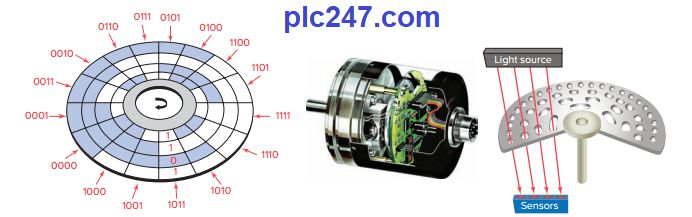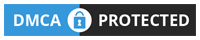PLC Guides

# Data types used in PLC (Number)Written by

Using PLCs requires us to become familiar with other number systems besides decimal. Some PLC models and individual PLC functions use
other numbering systems. This chapter deals with some of these numbering systems, including binary, octal, hexadecimal, BCD, Gray, and ASCII codes. The basics of each system, as well as conversion from one system to another, are
explained.

#### 1. Decimal System

The decimal system, which is most common to us, has a base of 10. The radix or base of a number system determines the total number of different symbols or digits
used by that system. For instance, in the decimal system, 10 unique numbers or digits梚.e., the digits 0 through 9? are used: the total number of symbols is the same as the base, and the symbol with the largest value is 1 less than the base.

#### 2. Binary System

The binary system uses the number 2 as the base. The only allowable digits are 0 and 1. With digital circuits it is easy to distinguish between two voltage levels (i.e., +5 V and 0 V), which can be related to the binary digits 1 and 0. Therefore, the binary system can be applied quite easily to PLCs and computer systems.

#### 3. Negative Numbers

If a decimal number is positive, it has a plus sign, if a number is negative, it has a minus sign. In binary number systems, such as used in a PLC, it is not possible to use
positive and negative symbols to represent the polarity of a number. One method of representing a binary number as either a positive or negative value is to use an extra digit, or sign bit, at the MSB side of the number. In the sign bit position, a 0 indicates that the number is positive, and a 1 indicates a negative number.

#### 4. Octal System

To express the number in the binary system requires many more digits than in the decimal system. Too many binary digits can become cumbersome to read or write.
To solve this problem, other related numbering systems are used.

The octal numbering system, a base 8 system, is used because 8 data bits make up a byte of information that can be addressed. Octal is a convenient means of handling
large binary numbers.

The hexadecimal (hex) numbering system is used in programmable controllers because a word of data consists of 16 data bits, or two 8-bit bytes. The hexadecimal system is a base 16 system, with A to F used to represent decimal numbers 10 to 15

#### 6. Binary Coded Decimal (BCD) System

The binary coded decimal (BCD) system provides a convenient way of handling large numbers that need to be input to or output from a PLC. As you can see from looking at the various number systems, there is no easy way to go from binary to decimal and back.

The BCD system provides a means of converting a code readily handled
by humans (decimal) to a code readily handled by the equipment (binary).

#### Gray Code

The Gray code is a special type of binary code that does not use position weighting. In other words, each position does not have a definite weight. The Gray code is set up so that as we progress from one number to the next, only one bit changes. This can be quite confusing for counting circuits, but it is ideal for encoder circuits. For example, absolute encoders are position transducers that use the Gray code to determine angular position.